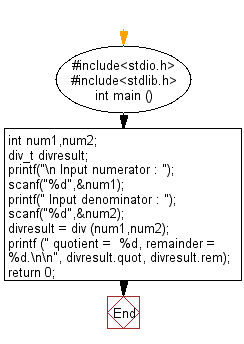﻿ C Program: Find the quotient and remainder of a division - w3resource# C Exercises: Find the quotient and remainder of a division

## C Variable Type : Exercise-10 with Solution

Write a C program to find the quotient and remainder of a division.

Sample Solution:

C Code:

``````#include<stdio.h>
#include<stdlib.h>

int main ()
{
int num1,num2;
div_t divresult;
printf("\n Input numerator : ");
scanf("%d",&num1);
printf(" Input denominator : ");
scanf("%d",&num2);

divresult = div (num1,num2);
printf (" quotient =  %d, remainder = %d.\n\n", divresult.quot, divresult.rem);
return 0;
}
```
```

Sample Output:

```Input numerator : 2000
Input denominator : 235
quotient =  8, remainder = 120.
```

Flowchart:C Programming Code Editor:

Improve this sample solution and post your code through Disqus

What is the difficulty level of this exercise?

Test your Programming skills with w3resource's quiz.

﻿

## C Programming: Tips of the Day

How I can print to stderr in C?

The syntax is almost the same as printf. With printf you give the string format and its contents i.e.:

`printf("my %s has %d chars\n", "string format", 30);`

With fprintf it is the same, except now you are also specifying the place to print to:

```File *myFile;
...
fprintf( myFile, "my %s has %d chars\n", "string format", 30);```

`fprintf( stderr, "my %s has %d chars\n", "string format", 30);`